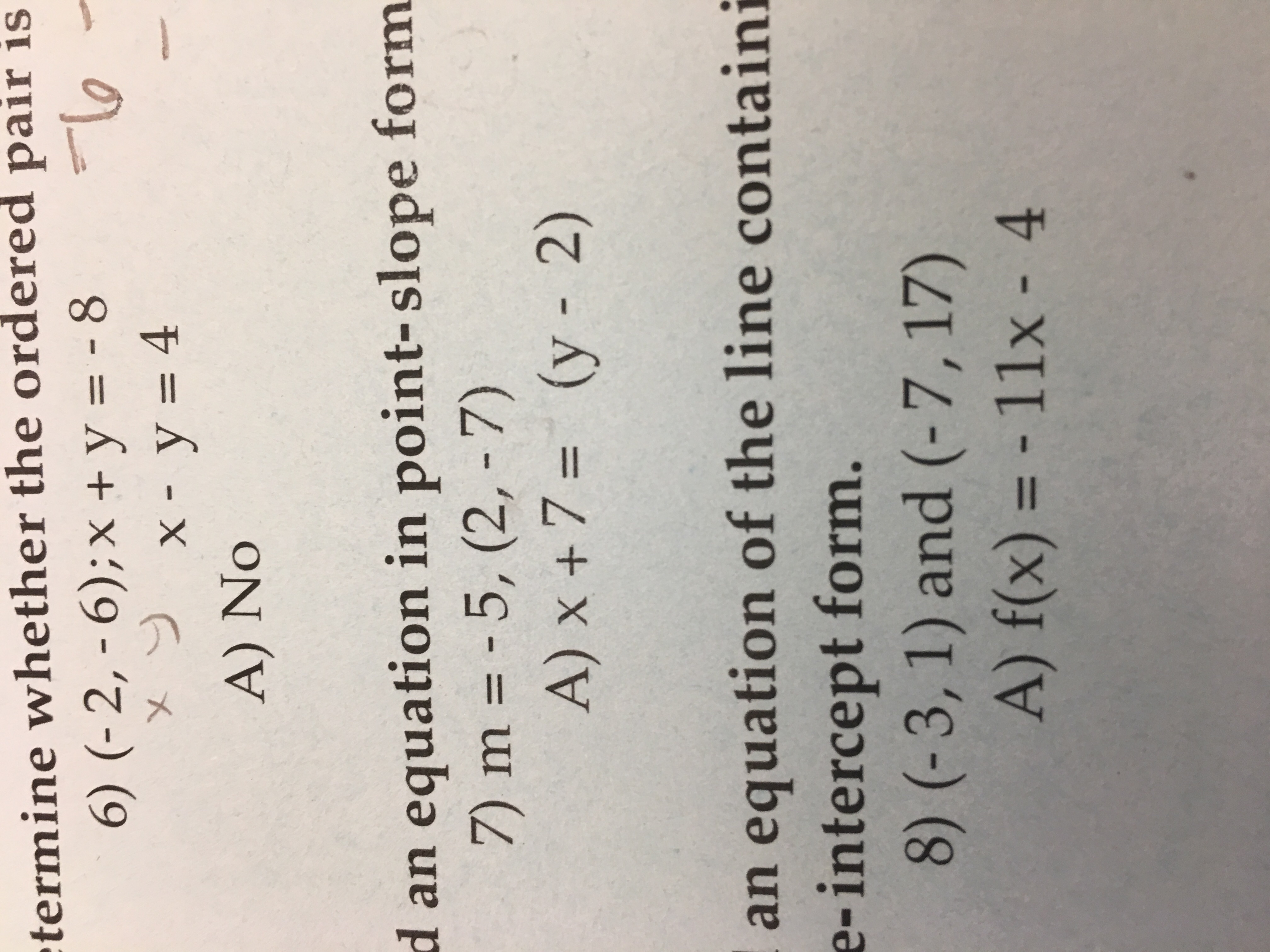termine whether the ordered pair isX.A) Noan equation in point-slope formd7)m=-5,(2,-7)(y-2)A) x + 7 =an equation of the line containie-interceptform.8) (-3, 1) and (-7,17)A) f(x) =-11x-4

Question

Find and equation in point intercept form of the the line having the specified slope and containing the point indicated

m=-5,(2,-7)help_outlineImage Transcriptionclosetermine whether the ordered pair is X. A) No an equation in point-slope form d 7)m=-5,(2,-7) (y-2) A) x + 7 = an equation of the line containi e-intercept form. 8) (-3, 1) and (-7,17) A) f(x) =-11x-4 fullscreen
Step 1

To calculate the equation in point intercept form where the slope of the line is -5 and the line passes through the point (2,-7). Now, first calculate equation of the line by using point slope form of the line then convert the equation in point intercept form.

Step 2

According to the point slope form of the line, if the line having slope m and passing through the point (x1,y1) then,

Step 3

The equation of line having slope -5 and the line passes through the p...

Want to see the full answer?

See Solution

Want to see this answer and more?

Our solutions are written by experts, many with advanced degrees, and available 24/7

See Solution
Tagged in

Algebra